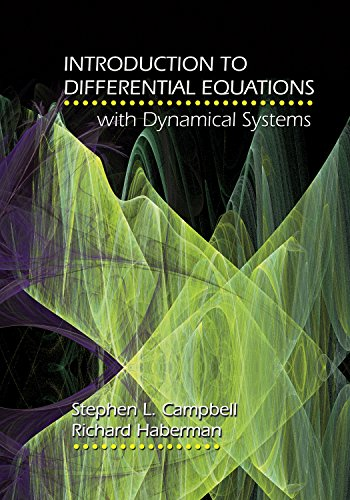Sale!

# Introduction to Differential Equations with Dynamical Systems 1st Edition Ebook PDF Version

N/A

### Introduction to Differential Equations with Dynamical Systems 1st EditionMany textbooks on differential equations are written to be interesting to the teacher rather than the student. Introduction to Differential Equations with Dynamical Systems is directed toward students. This concise and up-to-date textbook addresses the challenges that undergraduate mathematics, engineering, and science students experience during a first course on differential equations. And, while covering all the standard parts of the subject, the book emphasizes linear constant coefficient equations and applications, including the topics essential to engineering students. Stephen Campbell and Richard Haberman–using carefully worded derivations, elementary explanations, and examples, exercises, and figures rather than theorems and proofs–have written a book that makes learning and teaching differential equations easier and more relevant. The book also presents elementary dynamical systems in a unique and flexible way that is suitable for all courses, regardless of length.

ISBN 9780691124742

\$163.00 \$23.99Add to Wishlist
ISBN-13 9780691124742 N/A N/A N/A

### Introduction to Differential Equations with Dynamical Systems 1st EditionMany textbooks on differential equations are written to be interesting to the teacher rather than the student. Introduction to Differential Equations with Dynamical Systems is directed toward students. This concise and up-to-date textbook addresses the challenges that undergraduate mathematics, engineering, and science students experience during a first course on differential equations. And, while covering all the standard parts of the subject, the book emphasizes linear constant coefficient equations and applications, including the topics essential to engineering students. Stephen Campbell and Richard Haberman–using carefully worded derivations, elementary explanations, and examples, exercises, and figures rather than theorems and proofs–have written a book that makes learning and teaching differential equations easier and more relevant. The book also presents elementary dynamical systems in a unique and flexible way that is suitable for all courses, regardless of length.

ISBN 9780691124742

## Reviews

There are no reviews yet.